# DAV Class 7 Maths Chapter 13 Brain Teasers Solutions

The DAV Class 7 Maths Book Solutions and DAV Class 7 Maths Chapter 13 Brain Teasers Solutions of Symmetry offer comprehensive answers to textbook questions.

## DAV Class 7 Maths Ch 13 Brain Teasers Solutions

Question 1.
A. Tick (✓) the correct option:
(i) Mirror image of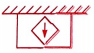is __________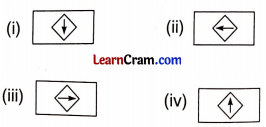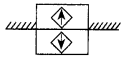Hence, (d) is the correct option.

(ii) How many lines of symmetry are there in the following figure?
(a) 1
(b) 2
(c) 3
(d) infinite
(d) infinite

A circle has infinite number of lines of symmetry.
Hence, (d) is the correct option.

(iii) How many lines of symmetry a scalene triangle has?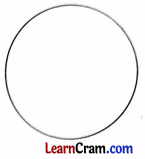(a) 3
(b) 2
(c) 1
(d) none
(d) none

Since a scalene triangle has no sides equal and no angles equal, so no lines of symmetry exist for it.
Hence, (d) is the correct option.

(iv) Which of the following letters has reflection symmetry about both horizontal and vertical mirrors ?
(a) O
(b) Y
(c) T
(d) L
(a) O

Letter ‘O’ has reflection symmetry about both horizontal and vertical mirrors.
Hence, (a) is the correct option.

(v) A regular heptagon has lines of symmetry.
(a) 5
(b) 6
(c) 7
(d) 8(c) 7

A regular heptagon has 7 lines of symmetry.
Hence, (c) is the correct option.(i) How many times can the following figure be folded so that it is symmetrical?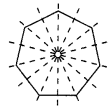Four times the figure can be folded so that it is symmetrical.

(ii) Complete the figure, so that the dotted line is the line of symmetry.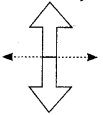(iii) Given the line of symmetry, find the other sides.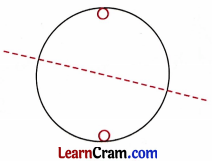(iv) Draw the line of symmetry in the following figure.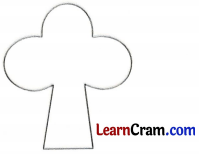(v) How many lines of symmetry are there in a regular pentagon and in a rectangle?A regular pentagon A rectangle (5 sides) has 5 lines of symmetry.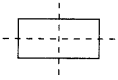A rectangle (opposite sides of symmetry. equal, all angles 90°) has 2 lines

Question 2.
How many lines of symmetry will the following have?
(i) A nine-sided regular polygon
Number of sides in this polygon is 9 it will have 9 lines of symmetry.

(ii) A ten-sided regular polygon
Number of sides in this polygon is 10, so it will have 10 lines of symmetry.

(iii) A circle
A circle has infinite number of lines of symmetry.

(iv) An equilateral triangle
An equilateral triangle has 3 lines of symmetry.

A quadrilateral has no line of symmetry as it is not a regular polygon.

(vi) A rhombus
A rhombus has 2 lines of symmetry.Question 3.
Draw the lines of symmetry of the following figures.Question 4.
Draw the lines of symmetry of the following figures.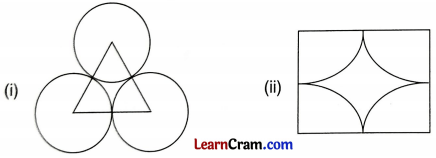Question 5.
In the following figures, the lines of symmetry are shown by the dotted lines. Find the other appropriate holes.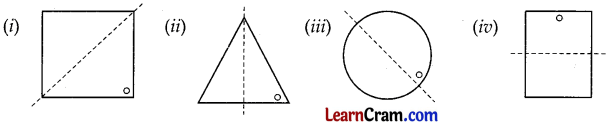Question 6.
Write 3 English alphabets each having.
(a) One line of symmetry(b) Two lines of symmetry(c) No line of symmetry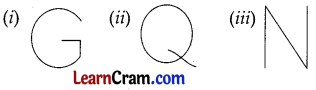Question 7.
l, m, n, t are the lines of symmetry of line segments XQ, PR, XY and RY respectively. If XP = 1.5 cm, find the length of the line segments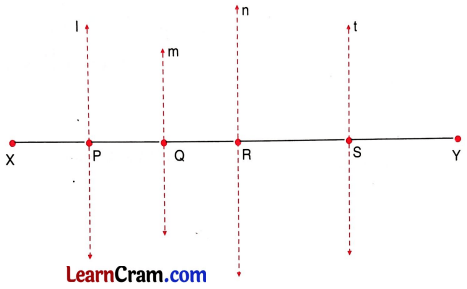(a) PQ
(a) XP = PQ (l is the line of symmetry of XQ).
∴ PQ = 1.5 cm

(b) QR
(b) PQ = QR (m is the line of symmetry of PR)
∴ QR = 1.5 cm

(c) RY
(c) RY = XR (x is the line of symmetry of XY)
∴ RY = 3×1.5 = 4.5cm

(d) PR
(d) PR = PQ + QR= 1.5 + 1.5 = 3 cm (m is the line of symmetry of PR).

Question 8.
If the dotted lines represent the lines of symmetry of the given angles, find the value of x.(i) x = $$\frac{90^{\circ}}{2}$$ = 45°
(ii) x = 2 × 85° = 170°Question 9.## DAV Class 7 Maths Chapter 14 Enrichment Questions

Question 1.
Mathematics in Architecture
Given here are a few of the finest examples of symmetry in architecture.Can you find more?
(i) India Gate at Delhi
(iii) Lotus Temple at Delhi
(iv) The White House at the United States
(v) Eiffel Tower at Paris
(vi) Humayun’s Tomb at Delhi
(vii) Ranakpur Jain Temple at Rajasthan

Question 2.
Tessellation
A tessellation is a simple process of tiling a floor or surface with a definite shape so that there is no overlapping or gaps.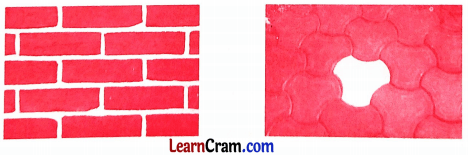Make tessellation pattern using:
(a) Hexagon
(b) Square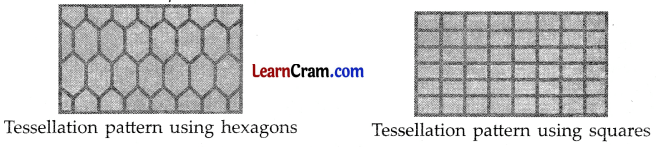Question 1.
Draw the lines of symmetry in the following figures.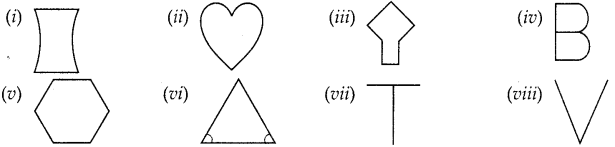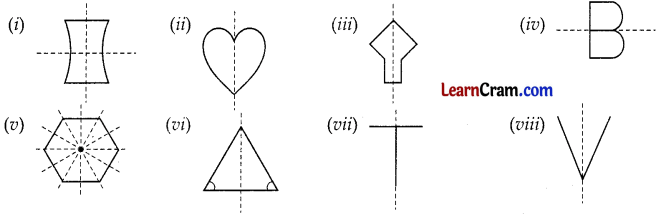Question 2.
Draw the figure for any 4 alphabet which have no line of symmetry.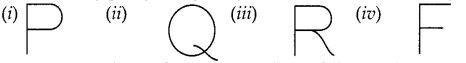Question 3.
How many lines of symmetry will the following have?
(i) Scalene triangle
Scalene triangle has no line of symmetry.

A quadrilateral has no line of symmetry, as it is not a regular figure.

(iii) A circle
A circle has infinite number of lines of symmetry.

(iv) A regular pentagon
A regular pentagon has 5 lines of symmetry.

(v) An equilateral triangle
An equilateral triangle has 3 lines of symmetry.

(vi) A regular hexagon
A regular hexagon has 6 lines of symmetry.

Question 4.
Fill in the blanks.
(i) A circle has __________ lines of symmetry.
infinite

(ii) A line has __________ lines of symmetry.
two

(iii) English alphabet having no lines of symmetry are __________.
Q, R, S, F, G, J, L, P, Z, N

(iv) Regular octagon has __________ number of lines of symmetry.
eight

(v) A quadrilateral has __________ lines of symmetry.
No

(vi) A square has __________ lines of symmetry.
4

(vii) An isosceles trapezium has __________ lines of symmetry.
one

(viii) English alphabet having only one line of symmetry are __________.
A, B, C, D, E, I, K, M, T, U, V, W, Y

(ix) An isosceles right triangle has __________ line of symmetry.
One

(x) Number of lines of symmetry of a regular polygon is equal to its number of __________
sides.

Question 5.
Identify the figures which have their lines of symmetry from the following figures.